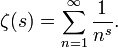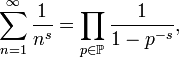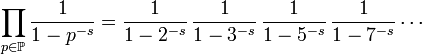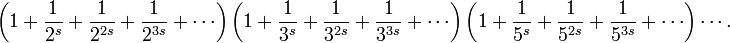# Prime Number Theorem

The list of prime numbers suggests that they thin out the further you go: 44% of the one-digit numbers are prime, but only 23% of the two-digit numbers and 16% of the three-digit numbers. The trial division method provides an intuitive explanation. To test whether a number n is prime, you have to try whether it can be divided by all numbers between 2 and$\sqrt n$. Large numbers have to undergo more tests, so fewer of them will be prime.

The Prime Number Theorem explains how fast the prime numbers thin out. It says that if you are looking around the number n, about one in every logn numbers is prime (here, logn denotes the natural logarithm of n). The formal statement of the Prime Number Theorem is$\lim_{x\to\infty} \frac{\pi(x) \log x}{x} = 1$

where π(x) denotes the number of primes$\le x$. This result was demonstrated independently by Jacques Hadamard and Charles de la Vallée Poussin in 1896. An essential part of their proof is the function$\zeta(s) = \sum_{n = 1}^{\infty} \frac{1}{n^s}.$

This function was already considered by the 18th century Swiss mathematician Leonhard Euler, who realized that$\sum_{n = 1}^{\infty} \frac{1}{n^s} = \prod_{p \in \mathbb{P}} \frac{1}{1 - p^{-s}},$

where the product on the right goes over all prime numbers:$\prod_{p \in \mathbb{P}} \frac{1}{1 - p^{-s}} = \frac{1}{1-2^{-s}} \, \frac{1}{1-3^{-s}} \, \frac{1}{1-5^{-s}} \, \frac{1}{1-7^{-s}} \cdots$

To see why this is so, we use the formula for the sum of a geometric series to write the product as$\left( 1 + \frac1{2^s} + \frac1{2^{2s}} + \frac1{2^{3s}} + \cdots \right) \left( 1 + \frac1{3^s} + \frac1{3^{2s}} + \frac1{3^{3s}} + \cdots \right) \left( 1 + \frac1{5^s} + \frac1{5^{2s}} + \frac1{5^{3s}} + \cdots \right) \cdots .$

Multiplying out this product, we get a sum of terms of the form$\frac{1}{(p_1^{a_1} p_2^{a_2} \cdots p_k^{a_k})^s},$

where p1, ..., pk are prime numbers and a1, ..., ak are positive integers. Euler's formula equating the sum and the product now follows from the fact that every number has a unique factorization into primes. This also indicates the connection between the function ζ and the prime numbers.

Euler used his formula to give an alternative proof that there are infinitely many primes. If we take s = 1, we get$\sum_{n = 1}^{\infty} \frac{1}{n} = \prod_{p \in \mathbb{P}} \frac{1}{1 - \frac1p}.$

The sum on the left is the harmonic series, which diverges. If the number of primes were finite, then the product would evaluate to a finite number. This contradiction shows that there are infinitely many primes.

The above definition for the function ζ only works when s > 1, because the infinite sum diverges otherwise, but a technique called analytic continuation can be used to extend the definition to all values of s, including complex numbers. The function with the extended domain is known as the Riemann zeta function. Hadamard and de la Vallée Poussin proved that this function cannot be zero in certain parts of the complex plane and used this to establish the prime number theorem. A proof not relying on complex analysis proved elusive, even though weaker results on the distribution of prime numbers have long been known. It was only in 1949 that Atle Selberg and Paul Erdős were able to establish the theorem by elementary means.

The location of the values s for which ζ(s) is zero is one of the biggest mysteries in contemporary mathematics. The Riemann hypothesis states all the zeros of the Riemann zeta function lie on two lines in the complex plane. A proof of the Riemann hypothesis would lead immediately to more precise estimates on how fast the function π(n) grows.

1. Ribenboim, Introduction to Analytic Number theory
2. Scharlau and Opolka, From Fermat to MinkowskiSome content on this page may previously have appeared on Citizendium.